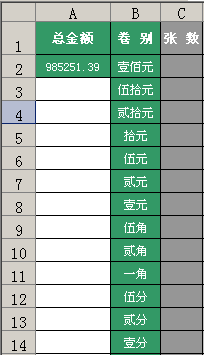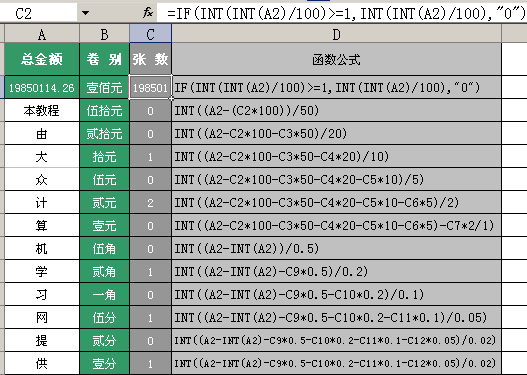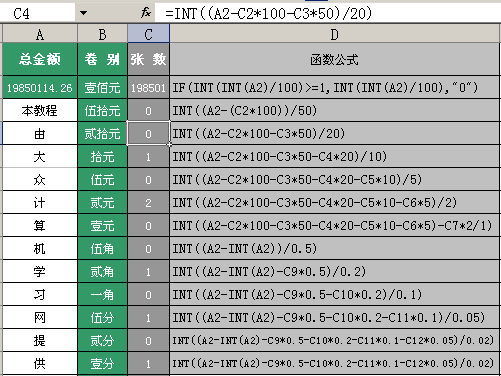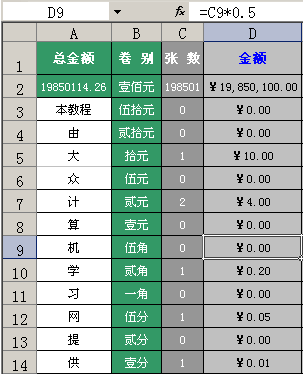# Excel根据金额求出壹佰、伍拾、贰拾、拾元、伍元、贰元、壹元、伍角等有几张

2013-08-10 16:31 阅读 513 次 评论关闭上表中，A2给出一个金额数，然后，在B列给出金额的不同币种，C列算出各由多少张的壹佰元、伍拾元、贰拾元、拾元、伍元贰元壹元伍角贰角一角伍分贰分壹分组成。使用公式虽然不复杂，但是却也不是那么的简单，为方便大家的学习，上图已增加了一辅助列，分别列出C列各单元的公式了，请你仔细查看，另外，为方便你的学习，再将公式写至下面：

IF(INT(INT(A2)/100)>=1,INT(INT(A2)/100),"0")
INT((A2-(C2*100))/50)
INT((A2-C2*100-C3*50)/20)
INT((A2-C2*100-C3*50-C4*20)/10)
INT((A2-C2*100-C3*50-C4*20-C5*10)/5)
INT((A2-C2*100-C3*50-C4*20-C5*10-C6*5)/2)
INT((A2-C2*100-C3*50-C4*20-C5*10-C6*5)-C7*2/1)
INT((A2-INT(A2))/0.5)
INT((A2-INT(A2)-C9*0.5)/0.2)
INT((A2-INT(A2)-C9*0.5-C10*0.2)/0.1)
INT((A2-INT(A2)-C9*0.5-C10*0.2-C11*0.1)/0.05)
INT((A2-INT(A2)-C9*0.5-C10*0.2-C11*0.1-C12*0.05)/0.02)
INT((A2-INT(A2)-C9*0.5-C10*0.2-C11*0.1-C12*0.05)/0.02)最后，再来查看一下，如何计算出角的公式：看上图吧。如果你全部都计算出正确的张数了，还可以增加一个各币种的总金额，如上图，希望本文对你有帮助。